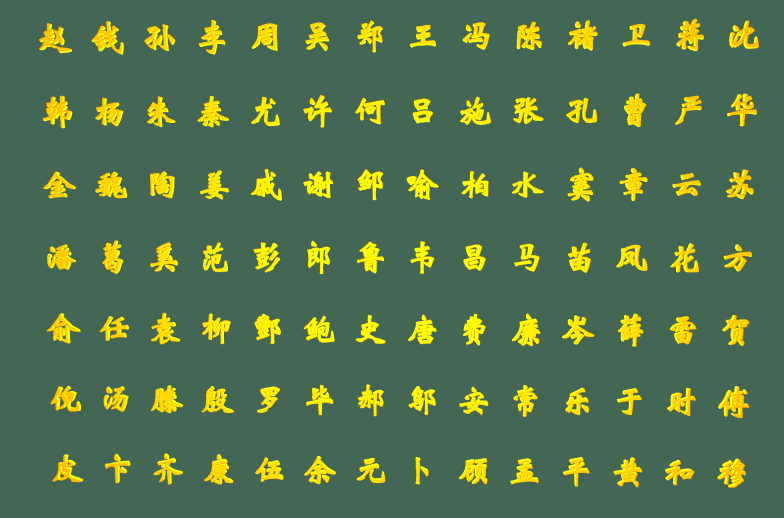# three.js顯示中文字體與tween應用詳析

## 2. tween操作文字動畫### 1.設置點的位置和朝向

```let vertices1 = new THREE.PlaneGeometry(1400, 900, 13, 6).vertices;
```

`d.position.clone().add(Vector3(0, 0, 1)) //這裡的d.position就是每個點的位置`

```let vertices2 = new THREE.SphereGeometry(500, 12, 9).vertices;
```

```for(let i=0; i<98; i++) {
let x = Math.sin(Math.PI / 12 * i) * 400;
let y = (49 - i) * 8;
let z = Math.cos(Math.PI / 12 * i) * 400;
positions3.push(new THREE.Vector3(x,y,z));
}```

`new THREE.Vector3(0, d.position.y, 0) //d.position.y就是點的y坐標`

### 2.tween動畫

```initTween() {
var pos = { time: 0 };
tween1 = new TWEEN.Tween(pos).to({ time: 1 }, 1000);
tween2 = new TWEEN.Tween(pos).to({ time: 2 }, 1000);
tween3 = new TWEEN.Tween(pos).to({ time: 0 }, 1000);
tween1.easing(TWEEN.Easing.Linear.None);
tween2.easing(TWEEN.Easing.Linear.None);
tween3.easing(TWEEN.Easing.Linear.None);
tween1.onUpdate(onUpdate);
tween2.onUpdate(onUpdate);
tween3.onUpdate(onUpdate);
}``````function onUpdate() {
let time = this._object.time; //動畫時刻值
if(flag == 0) {//這裡有三段動畫flag判斷是那一段動畫
group.children.forEach((d, i) => {//group裡面包含著所有文字網格
d.position.copy(positions3[i].clone().multiplyScalar(time / 2).add(positions1[i].clone().multiplyScalar(1 - time / 2)));
d.lookAt((new THREE.Vector3(0, d.position.y, 0).multiplyScalar(time / 2)).add(d.position.clone().add(lookAt1).multiplyScalar(1 - time / 2)));
})
} else if(flag == 1) {
group.children.forEach((d, i) => {
d.position.copy(positions1[i].clone().multiplyScalar(1 - time).add(positions2[i].clone().multiplyScalar(time)));
d.lookAt((d.position.clone().add(lookAt1).multiplyScalar(1 - time)).add(lookAt2.multiplyScalar(time)));
})
} else if(flag == 2) {
group.children.forEach((d, i) => {
d.position.copy(positions2[i].clone().multiplyScalar(2 - time).add(positions3[i].clone().multiplyScalar(time - 1)));
d.lookAt((lookAt2.multiplyScalar(2 - time)).add(new THREE.Vector3(0, d.position.y, 0).multiplyScalar(time - 1)));
})
}
}```

```click() {
tween1.stop();
tween2.stop();
tween3.stop();
if(flag == 0) {
tween1.start();
} else if(flag == 1) {
tween2.start();
} else if(flag == 2) {
tween3.start();
}
flag = (flag + 1) % 3;
},```